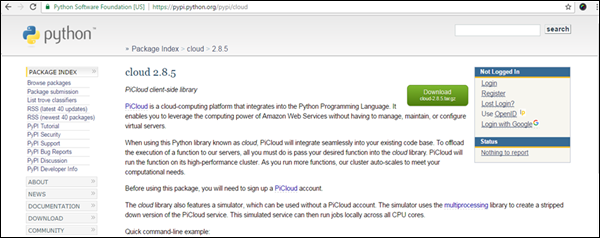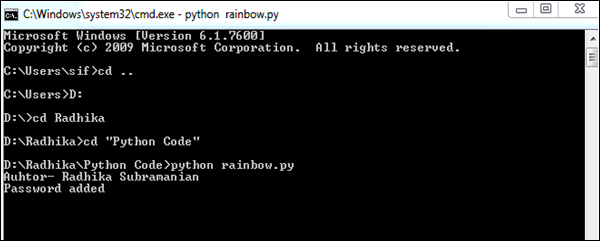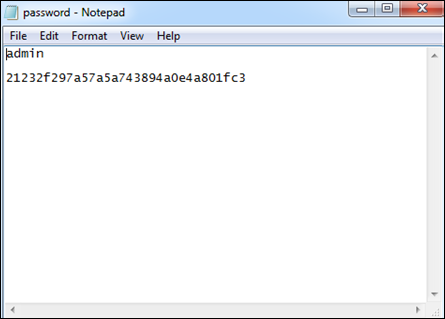# Python Forensics - Implementation of Cloud

Cloud computing can be defined as a collection of hosted services provided to users over the Internet. It enables organizations to consume or even compute the resource, which includes Virtual Machines (VMs), storage, or an application as a utility.

One of the most important advantages of building applications in Python programming language is that it includes the ability to deploy applications virtually on any platform, which includes cloud as well. It implies that Python can be executed on cloud servers and can also be launched on handy devices such as desktop, tablet, or smartphone.

One of the interesting perspectives is creating a cloud base with the generation of Rainbow tables. It helps in integrating single and multiprocessing versions of the application, which requires some considerations.

## Pi Cloud

Pi Cloud is the cloud computing platform, which integrates Python programming language with the computing power of Amazon Web Services.Let’s take a look at an example of implementing Pi clouds with rainbow tables.

## Rainbow Tables

A rainbow table is defined as a listing of all possible plain text permutations of encrypted passwords specific to a given hash algorithm.

• Rainbow tables follow a standard pattern, which creates a list of hashed passwords.

• A text file is used to generate passwords, which include characters or plain text of passwords to be encrypted.

• The file is used by Pi cloud, which calls the main function to be stored.

• The output of hashed passwords is stored in the text file as well.

This algorithm can be used to save passwords in the database as well and have a backup storage in the cloud system.

The following in-built program creates a list of encrypted passwords in a text file.

### Example

```import os
import random
import hashlib
import string
import enchant    #Rainbow tables with enchant
import cloud      #importing pi-cloud

def randomword(length):
return ''.join(random.choice(string.lowercase) for i in range(length))

def mainroutine():
engdict = enchant.Dict("en_US")

# Capture the values from the text file named password
while True:
randomword0 = randomword(6)
if engdict.check(randomword0) == True:
randomkey0 = randomword0+str(random.randint(0,99))
elif engdict.check(randomword0) == False:
englist = engdict.suggest(randomword0)
if len(englist) > 0:
randomkey0 = englist+str(random.randint(0,99))
else:
randomkey0 = randomword0+str(random.randint(0,99))

randomword3 = randomword(5)
if engdict.check(randomword3) == True:
randomkey3 = randomword3+str(random.randint(0,99))
elif engdict.check(randomword3) == False:
englist = engdict.suggest(randomword3)
if len(englist) > 0:
randomkey3 = englist+str(random.randint(0,99))
else:
randomkey3 = randomword3+str(random.randint(0,99))

if 'randomkey0' and 'randomkey3' and 'randomkey1' in locals():
whasher0 = hashlib.new("md5")
whasher0.update(randomkey0)
whasher3 = hashlib.new("md5")
whasher3.update(randomkey3)
whasher1 = hashlib.new("md5")
whasher1.update(randomkey1)
print(randomkey0+" + "+str(whasher0.hexdigest())+"\n")
print(randomkey3+" + "+str(whasher3.hexdigest())+"\n")
print(randomkey1+" + "+str(whasher1.hexdigest())+"\n")
fileb.write(randomkey0+" + "+str(whasher0.hexdigest())+"\n")
fileb.write(randomkey3+" + "+str(whasher3.hexdigest())+"\n")
fileb.write(randomkey1+" + "+str(whasher1.hexdigest())+"\n")

jid = cloud.call(randomword)  #square(3) evaluated on PiCloud
cloud.result(jid)
mainroutine()
```

### Output

This code will produce the following output −The passwords are stored in the text files, which is visible, as shown in the following screenshot.